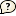Getting started Search Manifold recognizer Example

ε - invariantpolinomial of ε t(M) = ε + estimates of its real value ≤ t(M) ≤
GeometryE3 S3 S2 x R H2 x R SL2R Nil Sol H3 Volume ≤ V(M) ≤ Nongeometric
Complexityexact value of the complexity c(M)= 0 1 2 3 4 5 6 7 8 9 10 11 12 estimates of the complexity 0 1 2 3 4 5 6 7 8 9 10 11 12 ≤ c(M) ≤ 0 1 2 3 4 5 6 7 8 9 10 11 12
Homology groupNumber of summands in torsion subgroup Tor(H1) = 0 1 2 3 4 5 6 7 8 9 10 Torsion-free rank of H1 = 0 1 2 3 4 5 6 7 8 9 10 H1 = 0
Heegaard genusg(M) =
Stalling manifoldsSearch stallings manifold.
Seifert manifoldsEuler characteristic of base: Ď‡(F)= Number of exceptional fibers: n = Any 0 1 2 3 4 5 6 Orientation of F: Any Orientable Nonorientable
Search Reset